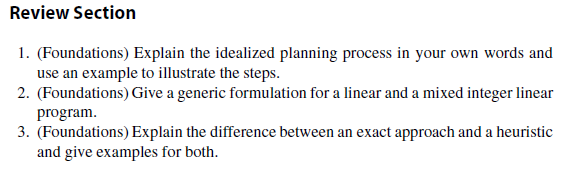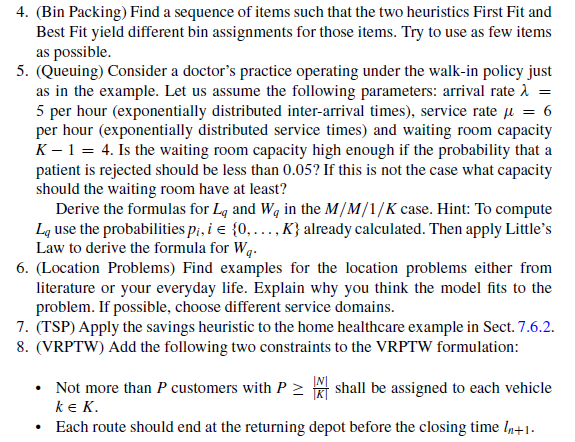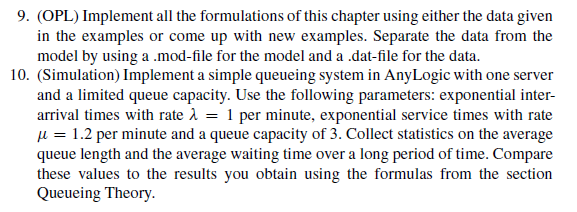# Service Optimization

Summary
This chapter provides an overview of Operations Research and its mathematical models for planning problems arising in the area of services. For a better understanding, a basic introduction into the field of Operations Research is given. Different examples from service areas are presented. Several methods for solving the mathematical problems are discussed. In addition, the optimization software called IBM ILOG CPLEX Optimization Studio is presented that can be used to determine an optimal solution for a mathematical problem. The use of simulation in the area of Operations Research is also discussed and the software AnyLogic is used to provide examples.

```[itex]
<mrow>
<mn>2</mn><mo>+</mo><mn>2</mn>
<mo>=</mo><mn>4</mn>
</mrow>
[/itex]```

Review Section
1. (Foundations) Explain the idealized planning process in your own words and use an example to illustrate the steps.

2. (Foundations) Give a generic formulation for a linear and a mixed integer linear program.

3. (Foundations) Explain the difference between an exact approach and a heuristic and give examples for both.

4. (Bin Packing) Find a sequence of items such that the two heuristics First Fit and Best Fit yield different bin assignments for those items. Try to use as few items as possible.

5. (Queuing) Consider a doctor’s practice operating under the walk-in policy just as in the example. Let us assume the following parameters: arrival rate &lambda 5 per hour (exponentially distributed inter-arrival times), service rate  D 6 per hour (exponentially distributed service times) and waiting room capacity K  1 D 4. Is the waiting room capacity high enough if the probability that a patient is rejected should be less than 0:05? If this is not the case what capacity should the waiting room have at least? Derive the formulas for Lq and Wq in the M=M=1=K case. Hint: To compute Lq use the probabilities pi; i 2 f0; : : : ;Kg already calculated. Then apply Little’s Law to derive the formula for Wq.

6. (Location Problems) Find examples for the location problems either from literature or your everyday life. Explain why you think the model fits to the problem. If possible, choose different service domains.

7. (TSP) Apply the savings heuristic to the home healthcare example in Sect. 7.6.2.

8. (VRPTW) Add the following two constraints to the VRPTW formulation:
• NotmorethanP customers with P  jNj jKj shall be assigned to each vehicle k 2 K.
• Each route should end at the returning depot before the closing time lnC1.

9. (OPL) Implement all the formulations of this chapter using either the data given in the examples or come up with new examples. Separate the data from the model by using a .mod-file for the model and a .dat-file for the data.

10. (Simulation) Implement a simple queueing system in AnyLogic with one server and a limited queue capacity. Use the following parameters: exponential interarrival times with rate  D 1 per minute, exponential service times with rate D 1:2 per minute and a queue capacity of 3. Collect statistics on the average queue length and the average waiting time over a long period of time. Compare these values to the results you obtain using the formulas from the section Queueing Theory.